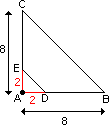## Definition Of Dilation

Dilation is a similarity transformation in which a figure is enlarged or reduced using a scale factor ? 0, without altering the center.

When the scale factor of a dilation is greater than 1, the dilation is an enlargement.
When the scale factor of a dilation is less than 1, the dilation is a reduction.

### Example of Dilation

In the figure shown, triangle ADE is enlarged to triangle ABC using a scale factor 4. Here, A is the center of dilation.A. 2
B. 5
C. 0.5
D. 0.2